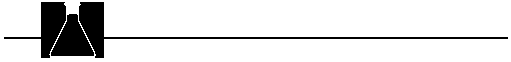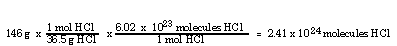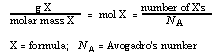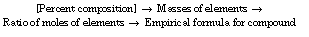## Tips For The Teachers

Problem Solving
1. Problem solving is essential to understanding the mole concept. It is important to illustrate a variety of permutations (moles to grams, volume of gas at STP to mass, molecules to moles, etc.) when solving mole problems. Practice should be given, initially with your assistance and later as independent assignments. After students master mole-mass and mass-mole conversions, proceed to percent composition problems. When percent composition problems are understood, the concept of empirical vs. molecular formula can be introduced and empirical formula problems can be solved. The determination of molecular formulas from empirical formulas and molar masses can be introduced either before or after problems dealing with calculating empirical formula.
2. In demonstrating how to solve mole-mass-entity problems, emphasize the relationship of mass and number of entities (molecules/atoms/formula units/ions, etc.) to moles. Suggest that students devise an equality that relates mole/ mass/molecules for the substance in the question. For example:
1 mol HCl = 36.5 g HCl = 6.02 x 10 23 molecules HCl

The mass (grams) of the substance can be converted to moles by using this relationship to construct a "unit conversion factor" and the unit-label method. Since mole is the unifying idea, if the number of molecules is desired and mass is given, mass should be converted to moles and then moles to molecules, using two factors (see transparency master).

Example: How many molecules are in 146 g HCl?3. If you prefer an algebraic approach, a generalized form of the relationship among mass, moles, and entities is:4. In percent composition problems, it is useful to illustrate the calculation using a compound having more than one atom of some element (e.g., K 2O) to illustrate that the total mass (2 x molar mass K atoms, not molar mass of K atoms) is used to calculate percent composition. It is a very common error to use the mass of 1 mol K (rather than 2 mol K) in calculating the percent K in K2O. The resulting mass percent potassium will be incorrect (too low by a factor of two). Remind students that the sum of the percents should be 100 (or close to 100).

5. One procedure for solving percent composition problems can be outlined using the mole concept:6. When illustrating empirical formula problems, start with a binary compound containing subscripts that are simple multiples of each other (e.g., CO2 , PCl3 , CH4). Then proceed to a binary compound where the mole relationship is more complex (2 to 3 or 3 to 4), requiring the additional step of multiplying by an integer to convert relative numbers of moles to integers. In solving empirical formula problems emphasize that subscripts give the relative numbers of moles of elements in the compound; if we know the relationship of the moles of the elements we know the subscripts. This type of problem is difficult for most students. Provide needed practice, both supervised and independent.

7. If students set up empirical formula problems "upside down" (i.e., divide atomic mass by mass of the element rather than calculate moles correctly), their subscripts will be opposite to what they should be (i.e., they will get the correct subscripts but for the wrong elements). Watch for this and reemphasize that students calculate moles of the elements. Of course, the units will be incorrect if students set up the problem this way, but that does not seem to bother many students. This would be a good place to emphasize how to use units to check that the problem solution is reasonable.

The procedure for solving empirical formula problems can be outlined using the mole concept: